# Objective

To study the relationship between the temperature of a hot body and its time of cooling by plotting a cooling curve.

# Theory

This Law of Cooling is named after the famous English Physicist Sir Isaac Newton, who conducted the first experiments on the nature of cooling.

## Statement of the Law :

According to Newton’s Law of Cooling, the rate of cooling of a body is directly proportional to the difference in temperatures of the body (T) and the surrounding (T0), provided difference in temperature should not exceed by 300C.

From the above statement,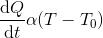For a body of mass m, specific heat s, and temperature T kept in surrounding of temperature T0;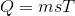Now, the rate of cooling,Hence ,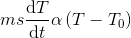Since the mass and the specific heat of the body are taken as constants, the rate of change of temperature with time can be written as,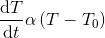The above equation explains that, as the time increases, the difference in temperatures of the body and surroundings decreases and hence, the rate of fall of temperature also decreases.

It can be graphically represented as,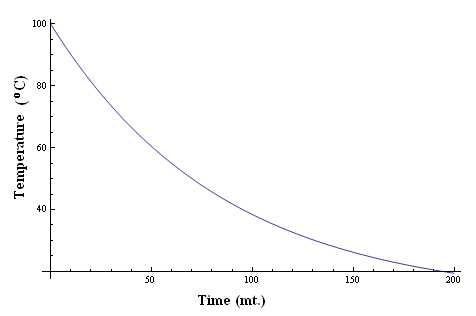# Learning Outcomes

• Students understand the different modes of transfer of heat.
• Students identify the variables which affect the cooling rate of a substance.
• Students verify Newton’s Law of Cooling.
• Students understand the relationship between temperature and time of cooling of objects.

Cite this Simulator: